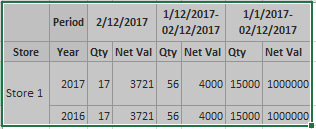# QlikView App Dev

Discussion Board for collaboration related to QlikView App Development.

Announcements
Our next Qlik Insider session will cover new key capabilities. Join us August 11th REGISTER TODAY
cancel
Showing results for
Did you mean:Contributor II

## Last Years same week day

Hello again everyone,

I have a pivot table with dimension the stores and expressions the daily quantity,month to date quanity, and year to date quantity.

The user selects the date from a listbox DD/MM/YYYY

What I want to achieve is to split the store's row into 3 subrows which the first will show current quantity ,the 2nd the perviou's year's same day quantity and the 3rd the comparisson of 1st and 2nd row.

I tried to calculate last years quantity but the result is the same as the current year.

The set analysis I use for the previous year is : Sum({\$< trn_date={"=\$(=Date(AddYears(max(trn_date),-1)))"}>} qty)

trn_date is the date field from which the user selects one date ,qty is the quantity.

What is the mistake I make? Please suggest a solution1 Solution

Accepted SolutionsMVP

Try this

=Pick(islandperiod,

Sum({<trn_date = {"\$(=TimeStamp(AddMonths(Max(trn_date), -12), 'YYYY-MM-DD hh:mm:ss.fff'))"}>} qty),

Sum({<trn_date = {">=\$(=MonthStart(Max(trn_date), -12)) <=\$(=AddMonths(Max(trn_date), -12))"}>} qty),

Sum({<trn_date = {">=\$(=YearStart(Max(trn_date), -1)) <=\$(=AddMonths(Max(trn_date), -12))"}>} qty)

)

7 RepliesContributor II
AuthorMVP

Would you be able to share a sample to look at this?Contributor II
Author

Here is a sample, and this is what i want to achieve is in the excel file.

Thank youMVP

Not sure how you got 2016 numbers? I don't see 12/04/2016... but you have 35 for that date? But if the data is correct, this should work

=Pick(islandperiod,

Sum({<trn_date = {">=\$(=MonthStart(Max(trn_date), -12)) <=\$(=AddMonths(Max(trn_date), -12))"}>} qty),

Sum({<trn_date = {">=\$(=YearStart(Max(trn_date), -1)) <=\$(=AddMonths(Max(trn_date), -12))"}>} qty)

)Contributor II
Author

This works fine for the month to date and year to date but for the exact same day last year returns 0 no matter which is the selected day.MVP

Try this

=Pick(islandperiod,

Sum({<trn_date = {"\$(=TimeStamp(AddMonths(Max(trn_date), -12), 'YYYY-MM-DD hh:mm:ss.fff'))"}>} qty),

Sum({<trn_date = {">=\$(=MonthStart(Max(trn_date), -12)) <=\$(=AddMonths(Max(trn_date), -12))"}>} qty),

Sum({<trn_date = {">=\$(=YearStart(Max(trn_date), -1)) <=\$(=AddMonths(Max(trn_date), -12))"}>} qty)

)Contributor II
Author

Perfect!Thank you so muchCommunity Browser